Processing ......FreeComputerBooks.com Links to Free Computer, Mathematics, Technical Books all over the World

Elementary Algebra
🌠 Top Free Data Science Books - 100% Free or Open Source!
• Title Elementary Algebra
• Author(s) Denny Burzynski, Wade Ellis
• Publisher: Saunders College Publishing 1989
• Hardcover/Paperback: 544 pages
• eBook: Mutiple Formats: PDF (904 pages, 10.3 MB), ePUB, Kindle, etc.
• Language: English
• ISBN-10: 0030294924
• ISBN-13: 9780030294921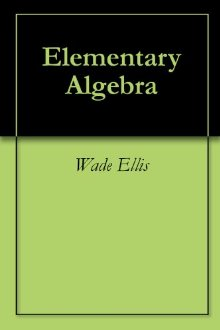Book Description

Elementary Algebra is a texbookt that covers the traditional topics studied in a modern elementary algebra course. It is intended for students who (1) have no exposure to elementary algebra, (2) have previously had an unpleasant experience with elementary algebra, or (3) need to review algebraic concepts and techniques.

• N/A
Reviews, Ratings, and Recommendations: Related Book Categories: Read and Download Links:Similar Books:
•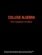College Algebra (Jay Abramson, et al.)

Written and reviewed by a team of highly experienced instructors, this book provides a comprehensive and multilayered exploration of algebraic principles. It is suitable for a typical introductory algebra course, and was developed to be used fexibly.

•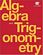Algebra and Trigonometry (Jay Abramson, et al)

This book provides a comprehensive and multi-layered exploration of algebraic principles. It is suitable for a typical introductory Algebra & Trigonometry course, and was developed to be used flexibly. The book meets the needs of a variety of programs.

•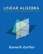Linear Algebra, Theory And Applications (Kenneth Kuttler)

This is a book on linear algebra and matrix theory. It gives a self- contained treatment of linear algebra with many of its most important applications which does not neglect arbitrary fields of scalars and the proofs of the theorems.

•Linear Algebra (Jim Hefferon)

This textbook covers linear systems and Gauss' method, vector spaces, linear maps and matrices, determinants, and eigenvectors and eigenvalues. Each chapter has three or four discussions of additional topics and applications.

•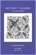Abstract Algebra: Theory and Applications (Thomas W Judson)

This book is designed to teach the principles and theory of abstract algebra to college juniors and seniors in a rigorous manner. Its strengths include a wide range of exercises, both computational and theoretical, plus many nontrivial applications.

•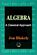Algebra: A Classical Approach (Jon Blakely)

This book provides a complete and contemporary perspective on classical polynomial algebra through the exploration of how it was developed and how it exists today, with discrete explanations from functions to quadratic and linear equations, etc.

•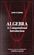Algebra: A Computational Introduction (John Scherk)

This book is a unique approach and presentation, the author demonstrates how software can be used as a problem-solving tool for algebra. It includes many computations, both as examples and as exercises.

•A Computational Introduction to Number Theory and Algebra

This introductory book emphasises algorithms and applications, such as cryptography and error correcting codes, and is accessible to a broad audience. It covers the basics of number theory, abstract algebra and discrete probability theory.

•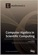Computer Algebra in Scientific Computing (Andreas Weber, et al.)

This book is dealing with a broad range of topics, ranging from algorithms, data structures, and implementation techniques for high-performance sparse multivariate polynomial arithmetic to computer algebra problems in quantum computing.

•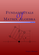Fundamentals of Matrix Algebra (Gregory Hartman)

A college (or advanced high school) level text dealing with the basic principles of matrix and linear algebra. It covers solving systems of linear equations, matrix arithmetic, the determinant, eigenvalues, and linear transformations.

•Matrix Algebra (Marco Taboga)

This is a course in matrix algebra, with a focus on concepts that are often used in probability and statistics. Matrix algebra is one of the most important areas of mathematics for data analysis and for statistical theory.

•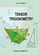Tensor Trigonometry (A.S. Ninul)

The tensor trigonometry is development of the flat scalar trigonometry from Leonard Euler classic forms into general multi-dimensional tensor forms with vector and scalar orthoprojections and with step by step increasing complexity and opportunities.

Book Categories
 :All CategoriesRecent BooksMiscellaneous BooksComputer LanguagesComputer ScienceData Science/DatabasesElectrical EngineeringJava and Java EE (J2EE)Linux and UnixMathematicsMicrosoft and .NETMobile ComputingNetworking and CommunicationsSoftware EngineeringSpecial TopicsWeb Programming
Other Categories